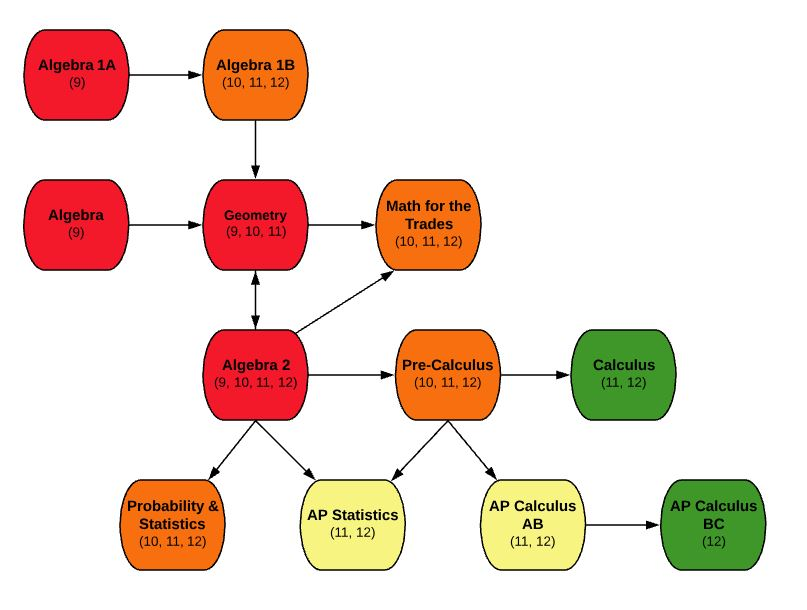EDIT MAIN

# MA700 AP Calculus ABCourse: MA700 AP Calculus AB

Subject Area: Advanced Placement, Articulated Credits, Mathematics

Credits: 1

Course Information:

This is a year-long course that requires selection of both semesters: S1 and S2. Accelerated College Advanced Program AP Calculus prepares students for more advanced study in mathematics, science, and other fields. The course begins with an extensive study of limits and uses this concept to develop differentiation and integration of polynomial, trigonometric, and logarithmic functions. An extensive study is conducted in the application areas of science and economics using differentiation and integration. This course will cover the equivalent of the first-semester college course. Students enrolling in this course are required to take the Advanced Placement Test for Calculus AB in May. Students are required to have a TI 83 or comparable calculator.

## Outcomes:

• Calculate limits graphically, algebraically, and numerically
• Find horizontal and vertical asymptotes
• Analyze continuity of a function
• Analyze instantaneous and average rate of change
• Understand the link between derivatives and instantaneous rate of change
• Use the appropriate method to calculate a derivative
• Use derivatives to calculate real-world rates of change and apply these derivatives appropriately
• Use derivatives to analyze a function
• Estimate the area under the curve with finite sums
• Understand the connection between antiderivatives and integrals
• Use the appropriate method to calculate an antiderivative
• Use antiderivatives to calculate volume and other applications

Prerequisites:

Minimum grade of C- in both MA414-S1 – Pre-Calculus S1 AND MA414-S2 – Pre-Calculus S2

Corequisites:

If you take this course, you must also take MA700-S2 – AP Calculus S2